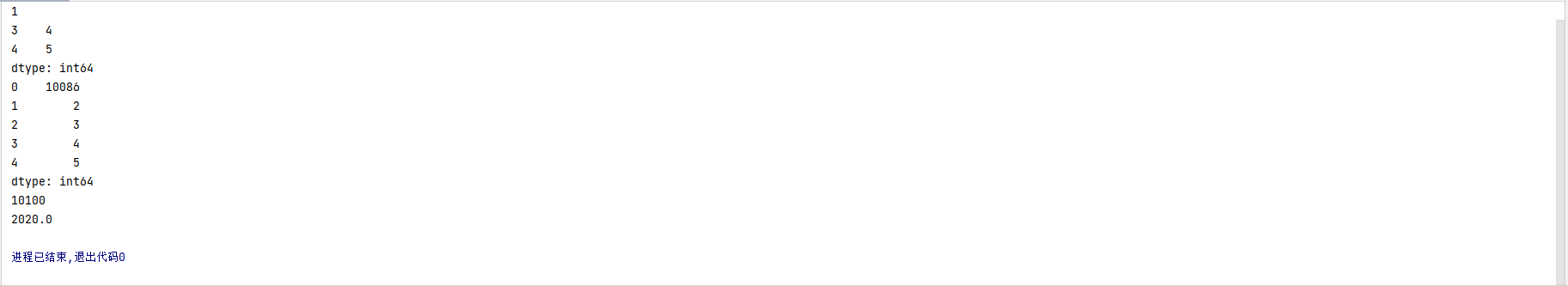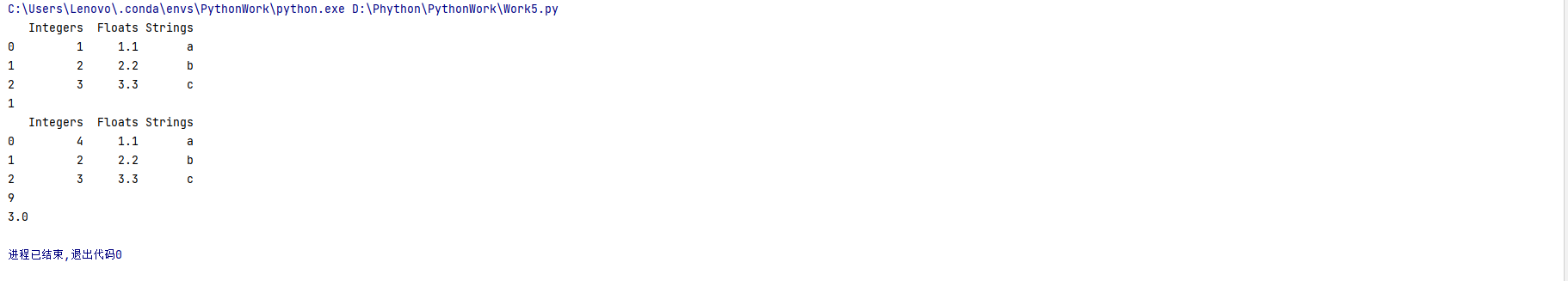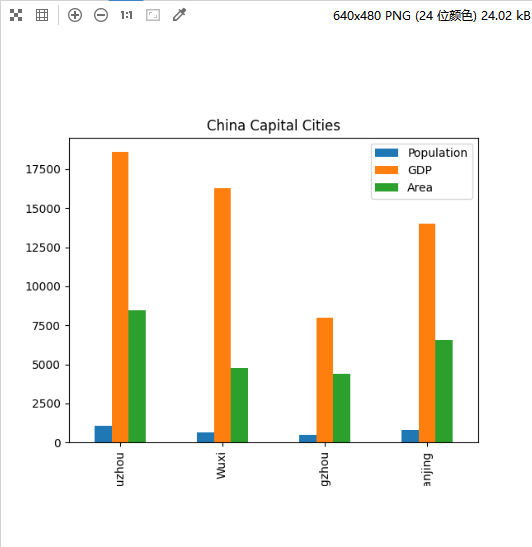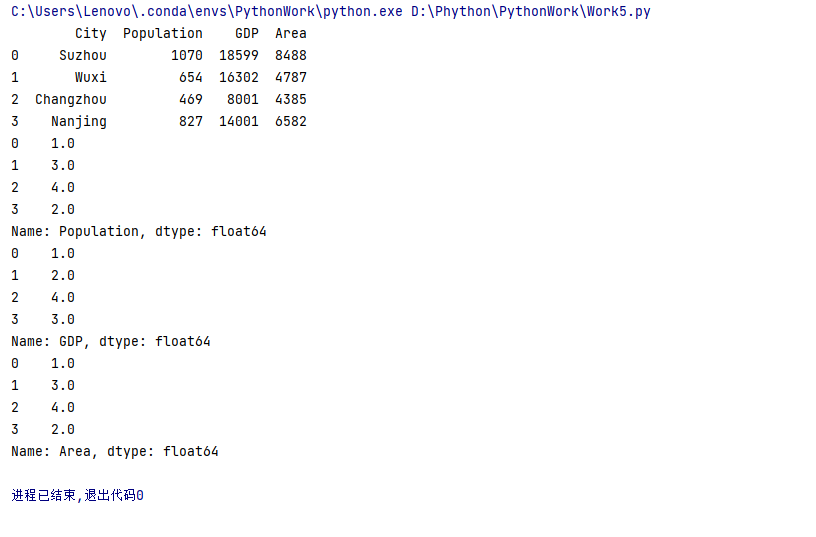# 2023（春）Python程序设计作业5：Pandas基础技能及综合应用

## 作业要求

1.    [基础要求]  基于Jupyter Notebook 完成以下实验一、实验二、实验三；
2.    [重点要求]  修改以下示例代码，以测试不同知识点。在博客上写出你：
•  修改的代码、
•  修改的愿意（意图）
•  代码运行的结果
•  你的结论

## 实验一：Series对象的应用

### 实验要求：

• 定义一个Series对象，包含5个整数数据；
• 访问、修改Series对象中的数据；
• 打印Series对象；
• 对Series对象进行计算，如求和、求平均值等
``````import pandas as pd

# 定义一个Series对象
s = pd.Series([1, 2, 3, 4, 5])

# 访问Series对象中的数据
print(s)  # 输出第一个元素
print(s[3:5])  # 输出第4个到第5个元素

# 修改Series对象中的数据
s = 10086

# 打印Series对象
print(s)

# 对Series对象进行计算
print(s.sum())  # 求和
print(s.mean())  # 求平均值``````## 实验二：DataFrame对象的应用

### 实验要求：

（1）定义一个DataFrame对象，包含3个列，每列分别为整数、浮点数和字符串类型；

（2）访问、修改DataFrame对象中的数据；

（3）对DataFrame对象进行计算，如求和、求平均值等。

（1）定义一个DataFrame对象，包含3个列，每列分别为整数、浮点数和字符串类型

``````data = {
'Integers': [1, 2, 3],
'Floats': [1.1, 2.2, 3.3],
'Strings': ['a', 'b', 'c']
}

df = pd.DataFrame(data)``````

（2）访问、修改DataFrame对象中的数据

``````value = df.iloc[0, 0]
print(value)

df.iloc[0, 0] = 4
print(df)``````

（3）对DataFrame对象进行计算，如求和、求平均值等。

``````sum = df['Integers'].sum()
print(sum)

mean = df['Integers'].mean()
print(mean)``````## 实验三：综合实例

### 实验要求：

（1）定义一个包含省会城市、人口、GDP、城市面积的DataFrame对象；

（2）计算各种排名，如人口最多的城市、GDP最高的城市等；

（3）使用Pandas绘图，可视化上述实验结果。

（1）定义一个包含省会城市、人口、GDP、城市面积的DataFrame对象

``````data = {
'City': ['Suzhou', 'Wuxi', 'Changzhou', 'Nanjing'],
'Population': [1070, 654, 469, 827],
'GDP': [18599, 16302, 8001, 14001],
'Area': [8488, 4787, 4385, 6582]
}

df = pd.DataFrame(data)``````

（2）计算各种排名，如人口最多的城市、GDP最高的城市等

``````# 计算各种排名
pop_rank = df['Population'].rank(ascending=False)
gdp_rank = df['GDP'].rank(ascending=False)
area_rank = df['Area'].rank(ascending=False)

print(pop_rank)
print(gdp_rank)
print(area_rank)``````

（3）使用Pandas绘图，可视化上述实验结果。

``````# 使用Pandas绘图，可视化实验结果
df.plot(kind='bar', x='City', y=['Population', 'GDP', 'Area'], title='China Capital Cities')
plt.show()``````...全文
6 回复 打赏 收藏 转发到动态 举报极简python入门课程
1、课程基于Python3讲解，从基础技能到实际案例，并辅以练习演示、课后作业及社区答疑；2、带你充分建立编程思维，扎实编码能力，学习后可独立完成小型程序开发；3、学会多线程等高级编程方法，编写出复杂的 Python 应用；4、通过标准库和第三方库，编写出能解决实际问题的工具；5、完整的 Python 入门+实战学习路径。2023Python程序设计作业1： 基础语法之控制流程

159发帖与我相关我的任务
python 高校 江苏省·南通市• 近7日
• 近30日
• 至今